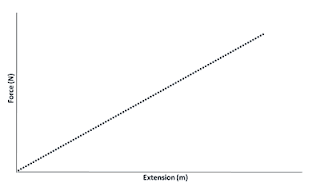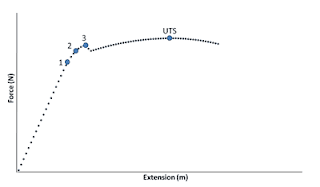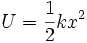## 2007-09-19

### Nota Fizik: ELASTICITY

Understanding Elasticity

Elasticity is the ability of a material to return to its original shape and size when the external force acting on it is removed.

It is due to the strong intermolecular forces between the molecules of the solid.
(you have to be able to explain elasticity in terms of intermolecular forces)

Hooke’s Law States that the extension of a spring is directly proportional to the applied force provided that the elastic limit is not exceeded.

Elastic limit of a spring is the maximum force that can be applied to a spring such that the spring will be able to be restored to its original length when the force is removed.

If the elastic limit is exceeded, the length of the spring is longer than the original length even though the force no longer acts on it. It is said to have permanent extension.

Hooke's law Graph
Force Vs Extensionk = force constant of the spring (equal of the gradient of the graph)
x = extension
Force constant is the force that is required to produce one unit of extension of the spring.It is the measure of the stiffness of the spring.The curve at the end occurs represents the moment before the material breaks.

Factors influencing the elasticity of a spring:

a. Type of spring material
b. diameter of the coil of spring
c. diameter of the wire of spring
d. arrangement of the spring.Point 1 is the Limit of Proportionality. Point 2 is the Elastic Limit. Point 3 is the Yield Point.

Before the limit of proportionality, the material obeys Hooke’s Law. After it, Force is no longer proportional to extension, and the graph begins to curve.

The Elastic Limit is the point when a material stops behaving elastically, and starts behaving plastically. The area before this point is called the elastic region; after it, the plastic region.

The Yield Point is the point where the material starts to stretch without applying any additional force.
Elastic Potential Energy, U

Elastic potential energy is the energy transferred to the spring when work is done on the spring.k = force constant
x = spring extension

Source: http://stuffaboutphysics.wordpress.com/2009/01/11/forceextension-graphs/